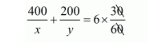# A man travels 600 km partly by train and partly by car.

Question:

A man travels 600 km partly by train and partly by car. If the covers 400 km by train and the rest by car, it takes him 6 hours 30 minutes. But, if the travels 200 km by train and the rest by car, he takes half an hour longer. Find the speed of the train and that of the car.

Solution:

Let the speed of the train be x km/hr that of the car be y km/hr, we have the following cases:

Case I: When a man travels 600Km by train and the rest by car

Time taken by a man to travel $400 \mathrm{Km}$ by train $=\frac{400}{x} h r s$

Time taken by a man to travel $(600-400)=200 \mathrm{Km}$ by car $=\frac{200}{y}$ hrs

Total time taken by a man to cover $600 \mathrm{Km}=\frac{400}{x}+\frac{200}{y}$

It is given that total time taken in 8 hours

$\frac{400}{x}+\frac{200}{y}=6 h r s 30 \mathrm{~min}$$\frac{400}{x}+\frac{200}{y}=6 \times \frac{1}{2}$

$\frac{400}{x}+\frac{200}{y}=\frac{13}{2}$

$200\left(\frac{2}{x}+\frac{1}{y}\right)=\frac{13}{2}$

$\left(\frac{2}{x}+\frac{1}{y}\right)=\frac{13}{2} \times \frac{1}{200}$

$\frac{2}{x}+\frac{1}{y}=\frac{13}{400} \cdots(i)$

Case II: When a man travels 200Km by train and the rest by car

Time taken by a man to travel $200 \mathrm{Km}$ by train $=\frac{200}{x} h r s$

Time taken by a man to travel $(600-200)=400 \mathrm{Km}$ by car $=\frac{400}{y} h r s$

In this case, total time of the journey in 6 hours 30 minutes + 30 minutes that is 7 hours,

$\frac{200}{x}+\frac{400}{y}=7$

$200\left(\frac{1}{x}+\frac{2}{y}\right)=7$

$\frac{1}{x}+\frac{2}{y}=\frac{7}{200}$..(ii)

Putting $\frac{1}{x}=u$ and,$\frac{1}{y}=u$, the equations ( $i$ ) and (ii) reduces to

$2 u+1 v=\frac{13}{400} \cdots(i i i)$

$1 u+2 v=\frac{7}{200} \cdots(v i)$

Multiplying equation (iii) by 6 the above system of equation becomes

$4 u+2 v=\frac{13}{200} \cdots(v)$

$1 u+2 v=\frac{7}{200} \cdots(v i)$

Substituting equationand, we get

$4 u+2 v=\frac{13}{200}$

$\frac{1 u+2 v=\frac{7}{200}}{3 u=\frac{6}{200}}$

$u=\frac{6}{200} \times \frac{1}{3}$

$u=\frac{2}{200}$

$u=\frac{1}{100}$

Putting $u=\frac{1}{100}$ in equation $(v i)$, we get

$1 u+2 v=\frac{7}{200}$

$\frac{1}{100}+2 v=\frac{7}{200}$

$2 v=\frac{7}{200}-\frac{1}{100}$

$2 v=\frac{7}{200}-\frac{2}{200}$

$2 v=\frac{7-2}{200}$

$2 v=\frac{5}{200}$

$v=\frac{5}{200} \times \frac{1}{2}$

$v=\frac{1}{80}$

Now

$u=\frac{1}{100}$

$\frac{1}{x}=\frac{1}{100}$

$x=100$

and

$\nu=\frac{1}{80}$

$\frac{1}{y}=\frac{1}{80}$

$y=80$

Hence, the speed of the train is $100 \mathrm{kn} / \mathrm{hr}$,

The speed of the car is $80 \mathrm{~km} / \mathrm{hr}$